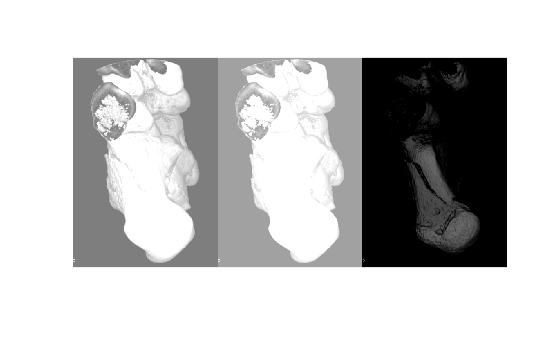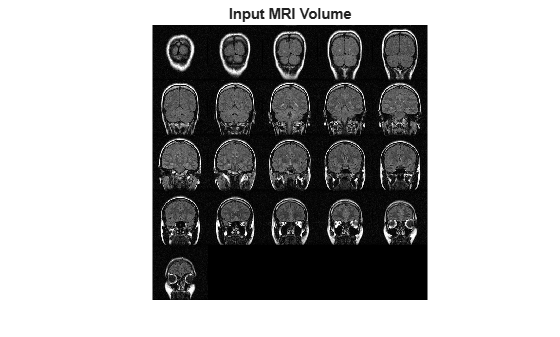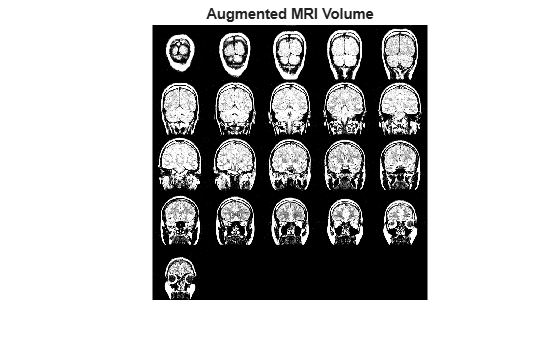# jitterIntensity

Randomly augment intensity of grayscale image or intensity volume

## Syntax

``J = jitterIntensity(I,Name=Value)``

## Description

You can use data augmentation to increase the variety and quantity of training data in deep learning applications, especially when available training data is limited, as is typical in medical imaging. Data augmentation can be intensity augmentation, geometric augmentation, or color augmentation. The `jitterIntensity` function performs intensity augmentation of grayscale images and intensity volumes by randomly augmenting their brightness, contrast, and gamma correction.

example

````J = jitterIntensity(I,Name=Value)` jitters the intensity of grayscale image or intensity volume `I` by randomly selecting brightness (shifting of intensity), contrast (scaling of intensity), and gamma correction values. To specify ranges for these values, use the corresponding name-value arguments.```

## Examples

collapse all

Import a grayscale CT image into the workspace. Crop the image to retain only the object of interest.

```I = dicomread("CT-MONO2-16-ankle.dcm"); I = imcrop(I,[101 51 290 420]);```

Randomly shift the intensity of the image `I` multiple times.

```J1 = jitterIntensity(im2single(I),Brightness=0.75); J2 = jitterIntensity(im2single(I),Brightness=0.75); J3 = jitterIntensity(im2single(I),Brightness=0.75);```

Visualize the augmented CT images to observe the impact of the randomly selected brightness value.

```figure montage({J1 J2 J3})```Load an MRI intensity volume into the workspace.

```load("mristack.mat","mristack"); V = mristack;```

Visualize the MRI volume.

```figure montage(V) title("Input MRI Volume")```Randomly shift and scale the intensity of the volume `V`.

`jitterV = jitterIntensity(V,Brightness=[-0.1 0.2],Contrast=3);`

Visualize the augmented MRI volume.

```figure montage(jitterV) title("Augmented MRI Volume")```Specify the location of a directory containing DICOM image files. Create a datastore for deep learning from the DICOM files.

```dicomDir = fullfile(matlabroot,"toolbox/images/imdata/dog"); dicomds = imageDatastore(dicomDir,FileExtensions=".dcm",ReadFcn=@(x)dicomread(x));```

Transform the datastore by gamma-correcting the intensity of the images with a random gamma value.

`jitterds = transform(dicomds,@(x)jitterIntensity(im2single(x),Gamma=[2 3]));`

Visualize the original and augmented datastore images.

```dicomImage = read(dicomds); jitterImage = read(jitterds); figure imshowpair(dicomImage,jitterImage,"montage")```## Input Arguments

collapse all

Grayscale image or intensity volume, specified as a 2-D numeric matrix or 3-D numeric array, respectively.

The function does not support 3-D RGB images and 4-D RGB volumes. For color augmentation of 3-D RGB images, see `jitterColorHSV`.

Data Types: `single` | `double` | `int16` | `uint8` | `uint16`

### Name-Value Arguments

Specify optional pairs of arguments as `Name1=Value1,...,NameN=ValueN`, where `Name` is the argument name and `Value` is the corresponding value. Name-value arguments must appear after other arguments, but the order of the pairs does not matter.

Example: `J = jitterIntensity(I,Brightness=0.75,Contrast=3,Gamma=[2 3])` augments the intensity of `I` by randomly selecting parameters from the ranges specified in the name-value arguments, and returns the augmented image `J`.

Brightness range, specified as a scalar in the range [0,1] or a two-element vector with elements in the range [–1, 1]. If you specify this value as a scalar, b, `jitterIntensity` shifts the intensity of the image or volume by a randomly selected value from the range [–b, b]. If you specify this value as a vector, [b1 b2], `jitterIntensity` shifts the intensity of the image or volume by a randomly selected value from the range [b1, b2]. You must specify values for b1 and b2 such that b2b1.

Example: `Brightness=0.75` shifts the intensity by a randomly selected value from the range [–0.75, 0.75].

Data Types: `single` | `double` | `int8` | `int16` | `int32` | `int64` | `uint8` | `uint16` | `uint32` | `uint64`

Contrast range, specified as a nonnegative scalar or two-element nonnegative vector. If you specify this value as a scalar, c, `jitterIntensity` scales the intensity of the image or volume by a randomly selected value from the range [min, 1+c], where min is the higher of 1–c and 0. If you specify the value as a vector, [c1 c2], `jitterIntensity` scales the intensity of the image or volume by a randomly selected value from the range [c1, c2].

Example: `Contrast=3` scales the intensity by a randomly selected value from the range [0, 4].

Data Types: `single` | `double` | `int8` | `int16` | `int32` | `int64` | `uint8` | `uint16` | `uint32` | `uint64`

Gamma range, specified as a nonnegative scalar or two-element nonnegative vector. If you specify the value as a scalar, g, `jitterIntensity` gamma-corrects the intensity of the image or volume with a randomly selected gamma value from the range [min, 1+g], where min is the higher of 1-g and 0. If you specify the value as a vector, [g1 g2], `jitterIntensity` gamma-corrects the intensity of the image or volume with a randomly selected gamma value from the range [g1, g2].

Example: `Gamma=[2 3]` gamma-corrects the intensity with a randomly selected gamma value from the range [2, 3].

Data Types: `single` | `double` | `int8` | `int16` | `int32` | `int64` | `uint8` | `uint16` | `uint32` | `uint64`

## Output Arguments

collapse all

Jittered image or volume, returned as a numeric matrix or array of the same size and data type as the input image or volume `I`. If the input image or volume `I` is of the data type single or double, the function rescales the intensities in `J` to the range [0, 1].

## Version History

Introduced in R2022b